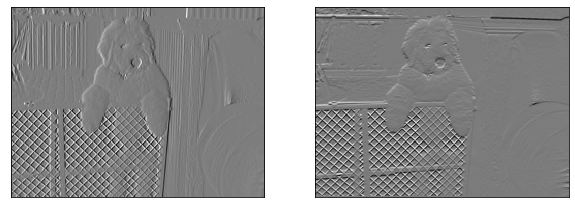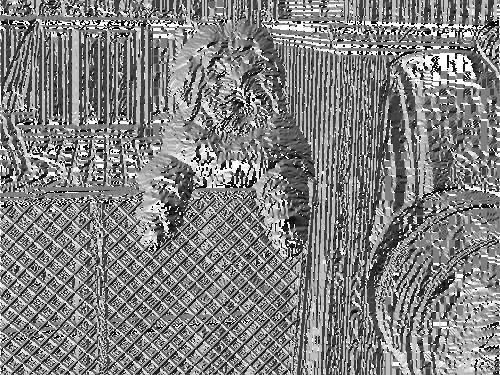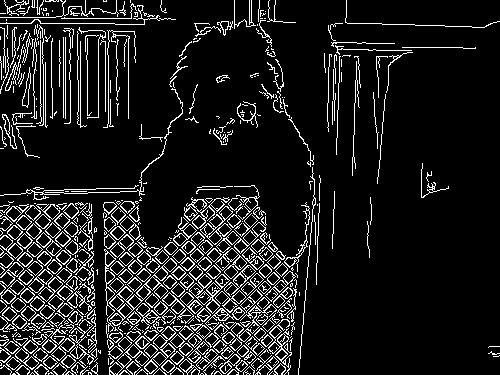# Edges Examples¶

:

from easycv import Image
from easycv.io.output import show_grid


For this example we will load an image from the Dog API. For more examples of image loading check Image examples.

:

img = Image("https://images.dog.ceo/breeds/sheepdog-english/n02105641_1045.jpg")
img

:To calculate the gradient of the image we simply apply the Gradient Transform for both axis, for more information check the reference. The gradients are displayed in a grid created using show_grid().

:

grad_x = img.apply(Gradient(axis="x"))To calculate the magnitude of the image gradient we simply apply the Gradient transform with no axis, for more information check the reference.

:

img.apply(Gradient())

::

img.apply(GradientAngle(size=1))

:## Canny Edge¶

To get the edges of an image we just apply the Canny transform with the low and high threshold, for more information check the reference.

:

img.apply(Canny(low=100, high=200))

: